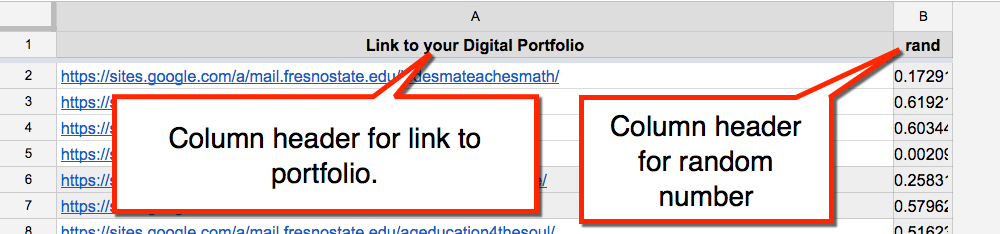Sometimes I have need to randomize the results from a Google Form. For example, I had students submit links to their digital portfolios. I want the other students to view the portfolios. However, if the results are not randomized the first few in the list will be viewed many times. The students at the bottom of the list will not be viewed at all.

Sorting by a dynamic random number causes the results to continuously sort randomly.

# Insert a Random Column

Insert a column into the Form results on the spreadsheet. Insert the column next to the column you wish to randomly sort.
Name the column “Random.”
In the cells type the formula =rand().
Each row of data will need to have a random number assigned.## Insert a Sheet

In row 1 type the header columns for the data you wish to sort and display. Include the random column. Freeze the first row to lock in place.# Sort Function

Use the =sort() function to sort the data from the Form responses tab. In cell A2 of the new sheet, type the formula:

=sort(‘Form Responses 1’!B:C,2,true)=sort(‘Tab Name’!Range, sort column, true)
The sort formula first lists the name of the tab where the data is being pulled from. The tab name is in single quotations. An exclamation mark follows the tab name. The range of data to be sorted comes next. Note that the range B:C says to sort everything in columns B to C. After the comma is the column you wish to sort by. In my data column B has the data and column C has the random number. I want to sort by random number. Column B is the 1st column in the range and column C is the 2nd column in the range. Thus, 2 is the sort column. True sorts ascending, False sorts descending.

# Hide Form Results

This leaves only the tab with the sorted information. Click here for a sample.

## Subscribe to the Alice Keeler Newsletter